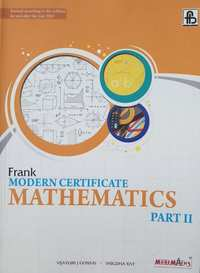Share

# Frank solutions for Class 10 Maths chapter 18 - Constructions

#### Frank Frank Class 10 Mathematics Part 2## Chapter 18: Constructions

Exercise 18.1

#### Frank solutions for Class 10 Maths Chapter 18 Exercise Exercise 18.1 [Page 0]

Draw a circle of radius 4 cm and take a point Pon its circumference. Construct a tangent to the circle at P.

Construct Δ ABC in which AB = 5 cm, BC = 4. 5 cm and ∠ ABC = 60" .. Construct a cirde to circumscribe. Δ ABC.

Using ruler and compasses only, construct a triangle ABC in which AB=S cm, BC=6 cm and CA=4.5 cm. Construct a circle passing through A, Band c.

Using ruler and compasses only, construct and equilateral triangle with side 4.5 cm. Draw a circumcircle of this triangle and measure its radius.

Using ruler and compasses only, construct  Δ ABC in which BC=7.5 cm, ∠ ABC = 60°  and AC - AB= 1.5 cm. Inscribe a circle in the Δ ABC and measure its radius.

Draw a circle with radius 3 cm and inscribe an equilateral triangle in it.

Draw a circle of radius 2. 5 cm and circumscribe a square about it.

Inscribe a regular hexagon in a circle of radius 3 .5 cm.

Inscribe a regular pentagon in a circle of radius 4 cm. Inscribe a circle in this pentagon and me a sure its radius.

Draw a line segment OA , 5 cm long. AT O , using ruler and compasses only, construct OB such that , ∠ AOB = 37.5° construct a circle to touch OA at A and to touch OB at B.

Draw a circle of radius 4.5 cm. Take a point Pon its circumference. Construct a tangent to the circle at P without using the centre.

Draw line segments OA = 4.5 cm, OB = 3.2 cm such that ∠ AOB = 45°. Construct a circle touching OA at A and passing through B.

Draw a circle with centre O and radius 2.5 cm. Take a point P at a distance of 6 cm from the centre. Using ruler and compasses only construct the tangents to the circle from the point P.

Draw a circle with centre O and radius 3 cm. Take a point P outside the circle. Draw tangents to the circle from P without using the centre and using only ruler and compasses.

Using ruler and compasses only, draw tangents to a circle of radius 3 cm from a point 5 cm from the centre. What is the length of each of them ?

Draw two circles of radii 2.5 cm and 3.5 cm respectively so that their centres are 8 cm apart. Draw direct comm on tangents to the circle.

Draw two circles of radii 3.5 cm and 2 cm respectively so that their centres are 6 cm apart. Draw direct common tangents to the circle and show that they are equal in length.

Draw two circles of radii 3 cm and 3.5 cm, their centres being 8 cm apart. Construct a transverse common tangent and measure its length.

Draw two circles with radii 2.5 cm and 4 cm and with their centres 7 cm apart.
Draw a direct common tangent and a transverse common tangent. Calculate the length of the direct common tangent.

## Chapter 18: Constructions

Exercise 18.1

#### Frank Frank Class 10 Mathematics Part 2## Frank solutions for Class 10 Mathematics chapter 18 - Constructions

Frank solutions for Class 10 Maths chapter 18 (Constructions) include all questions with solution and detail explanation. This will clear students doubts about any question and improve application skills while preparing for board exams. The detailed, step-by-step solutions will help you understand the concepts better and clear your confusions, if any. Shaalaa.com has the CISCE Frank Class 10 Mathematics Part 2 solutions in a manner that help students grasp basic concepts better and faster.

Further, we at Shaalaa.com provide such solutions so that students can prepare for written exams. Frank textbook solutions can be a core help for self-study and acts as a perfect self-help guidance for students.

Concepts covered in Class 10 Mathematics chapter 18 Constructions are Circumscribing and Inscribing a Circle on a Regular Hexagon, Circumscribing and Inscribing a Circle on a Triangle, Construction of Tangents to a Circle.

Using Frank Class 10 solutions Constructions exercise by students are an easy way to prepare for the exams, as they involve solutions arranged chapter-wise also page wise. The questions involved in Frank Solutions are important questions that can be asked in the final exam. Maximum students of CISCE Class 10 prefer Frank Textbook Solutions to score more in exam.

Get the free view of chapter 18 Constructions Class 10 extra questions for Maths and can use Shaalaa.com to keep it handy for your exam preparation

S